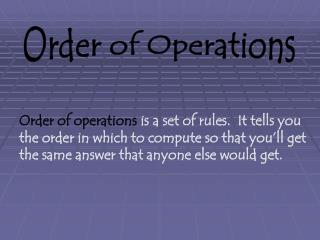Download PresentationOrder of Operations

# Order of Operations - PowerPoint PPT PresentationDownload Presentation## Order of Operations

- - - - - - - - - - - - - - - - - - - - - - - - - - - E N D - - - - - - - - - - - - - - - - - - - - - - - - - - -
##### Presentation Transcript

1. Order of Operations Order of operations is a set of rules. It tells you the order in which to compute so that you’ll get the same answer that anyone else would get.

2. Example: Compute 6 + 4 x 3 ÷ 6 = But, if you knew about order of operations, you’d do this. 6 + 4 x 3 ÷ 6 If you work in order from left to right, you’d do this first. 6 + 4 x 3 ÷ 6 = 12 10 6 + 12 ÷ 6 10 x 3 ÷ 6 2 30 6 + 2 = 8 30 ÷ 6 = 5

3. But, if you knew about order of operations, you’d do this. 6 + 4 x 3 ÷ 6 If you work in order from left to right, you’d do this first. 6 + 4 x 3 ÷ 6 = 12 10 6 + 12 ÷ 6 10 x 3 ÷ 6 2 30 6 + 2 = 8 30 ÷ 6 = 5  6 + 4 x 3 ÷ 6 really does equal 8. The order of operations makes sure that there is only one correct answer for this or any other computation.

4. Click on the computer for an Order of Operation mini lesson

5. Using Parentheses to Show Order of Operations Sometimes, when you write a problem, you don’t want to follow the regular order of operations. You can use parentheses to say do this first.

6. Each of 5 friends got a full box of snacks and an extra 6 snacks. Write an equation to show how many snacks are in all those boxes and all those extra snacks. (Each snack box has the same amount of snacks.) Example: represents a snack box

7. Each of 5 friends got a full box of snacks and an extra 6 snacks. Write an equation to show how many snacks are in all those boxes and all those extra snacks Even if you don’t know how many snacks are in a box, you can write an expression to show how many. 5 x + 6

8. 5 x + 6 The order of operations would tell you to multiply 5 by then add 6. But every friend has a sum of snacks ( + 6) and you want to multiply the sum by 5. Each of 5 friends got a full box of snacks and an extra 6 snacks. Write an equation to show how many snacks are in all those boxes and all those extra snacks

9. Use parentheses to group the sum: 5 x ( + 6). So, if = 4, you compute like this: The order of operations would tell you to multiply 5 by then add 6. But every friend has a sum of snacks ( + 6) and you want to multiply the sum b y 5.  5 x (4 + 6) 5 x 10 50 =

10. Rules for Order of Operations To make sure that everyone finds the same answer when computing, we have rules called order of operations. 1. Compute inside the parentheses. 2. Calculate the exponents and roots. Multiply or divide left to right. 3. 4. Add or Subtract left to right.

11. This silly sentence may help you remember: “Please excuse my dear Aunt Sally.” 1. Compute inside the parentheses. 2. Calculate the exponents and roots. Multiply or divide left to right. 3. Add or Subtract left to right. 4.

12. Set up the equation Example: 5 friends have collected 300 cans. They take them to the store that pays 6¢ each for aluminum cans. Fifteen of the cans are not aluminum. If they share the money equally, how much does each friend get? 300 cans, 15 of them worthless 300 - 15 Each aluminum can is worth 6¢ 6 x (300 – 15) Five friends share equally 6 x (300 – 15) ÷ 5

13. Follow the Order of Operations  Each friend will get 342¢ or \$3.42 for the cans. 6 x (300 – 15) ÷ 5 1. Compute inside parentheses 6 x (285) ÷ 5 2. Do the exponents or roots no exponents or roots 3. Multiply or divide left to right 6 x (285) = 1710 1710 ÷ 5 = 342

14. Practice 4 x (53 – 18) ÷ 5 = Please excuse my dear Aunt Sally Remember : 1. Compute inside the parentheses 4 x (53 – 18) ÷ 5 = 4 x 35 ÷ 5 =

15. Please excuse my dear Aunt Sally Remember : 4 x 35 ÷ 5 = 4 x (53 – 18) ÷ 5 = 2. Do the exponents or roots no exponents or roots 3. Multiply or divide left to right ÷ 5 = 140 28

16. Please excuse my dear Aunt Sally Remember : More Practice 16 ÷ 4 + (4 – 3) x 4 16 ÷ 4 + (4 – 3) x 4 16 ÷ 4 + 1 x 4 4 + 4 4 + 4 = 8